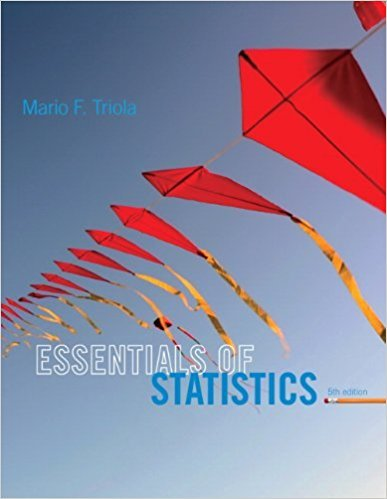×
×

# Solutions for Chapter 3.2: Measures of Center## Full solutions for Essentials of Statistics | 5th Edition

ISBN: 9780321924599Solutions for Chapter 3.2: Measures of Center

Solutions for Chapter 3.2
4 5 0 321 Reviews
12
3
##### ISBN: 9780321924599

Essentials of Statistics was written by and is associated to the ISBN: 9780321924599. Since 39 problems in chapter 3.2: Measures of Center have been answered, more than 15615 students have viewed full step-by-step solutions from this chapter. This textbook survival guide was created for the textbook: Essentials of Statistics, edition: 5. Chapter 3.2: Measures of Center includes 39 full step-by-step solutions. This expansive textbook survival guide covers the following chapters and their solutions.

Key Statistics Terms and definitions covered in this textbook
• a-error (or a-risk)

In hypothesis testing, an error incurred by failing to reject a null hypothesis when it is actually false (also called a type II error).

A formula used to determine the probability of the union of two (or more) events from the probabilities of the events and their intersection(s).

• Assignable cause

The portion of the variability in a set of observations that can be traced to speciic causes, such as operators, materials, or equipment. Also called a special cause.

• Bivariate normal distribution

The joint distribution of two normal random variables

• Central composite design (CCD)

A second-order response surface design in k variables consisting of a two-level factorial, 2k axial runs, and one or more center points. The two-level factorial portion of a CCD can be a fractional factorial design when k is large. The CCD is the most widely used design for itting a second-order model.

• Coeficient of determination

See R 2 .

• Components of variance

The individual components of the total variance that are attributable to speciic sources. This usually refers to the individual variance components arising from a random or mixed model analysis of variance.

• Conditional probability distribution

The distribution of a random variable given that the random experiment produces an outcome in an event. The given event might specify values for one or more other random variables

• Confounding

When a factorial experiment is run in blocks and the blocks are too small to contain a complete replicate of the experiment, one can run a fraction of the replicate in each block, but this results in losing information on some effects. These effects are linked with or confounded with the blocks. In general, when two factors are varied such that their individual effects cannot be determined separately, their effects are said to be confounded.

• Correction factor

A term used for the quantity ( / )( ) 1 1 2 n xi i n ? = that is subtracted from xi i n 2 ? =1 to give the corrected sum of squares deined as (/ ) ( ) 1 1 2 n xx i x i n ? = i ? . The correction factor can also be written as nx 2 .

• Correlation

In the most general usage, a measure of the interdependence among data. The concept may include more than two variables. The term is most commonly used in a narrow sense to express the relationship between quantitative variables or ranks.

• Defects-per-unit control chart

See U chart

• Discrete distribution

A probability distribution for a discrete random variable

• Discrete uniform random variable

A discrete random variable with a inite range and constant probability mass function.

• Empirical model

A model to relate a response to one or more regressors or factors that is developed from data obtained from the system.

• Error mean square

The error sum of squares divided by its number of degrees of freedom.

• Estimate (or point estimate)

The numerical value of a point estimator.

• Experiment

A series of tests in which changes are made to the system under study

• Exponential random variable

A series of tests in which changes are made to the system under study

• First-order model

A model that contains only irstorder terms. For example, the irst-order response surface model in two variables is y xx = + ?? ? ? 0 11 2 2 + + . A irst-order model is also called a main effects model

×